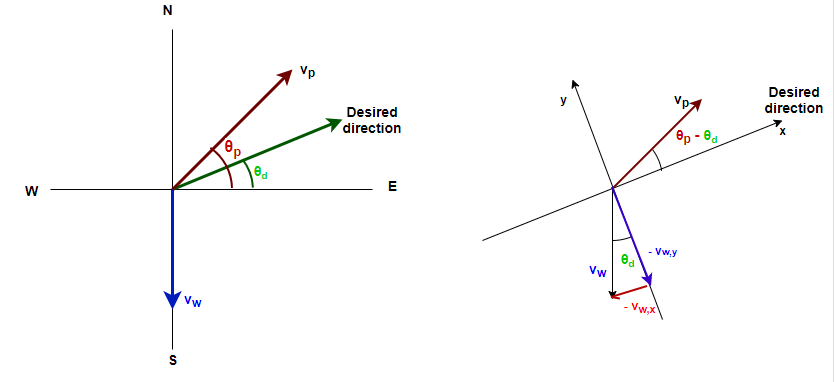# Problem: An airplane, whose air speed is 560 km/h, is supposed to fly in a straight path 36.0° N of E. But a steady 82 km/h wind is blowing from the north. In what direction should the plane head?

🤓 Based on our data, we think this question is relevant for Professor Rajeev's class at UR.

###### FREE Expert Solution

This problem requires us to determine the direction the plane should head so that it attains the desired direction.

Whenever given a problem about a plane and blowing wind, it's safe to assume it's a two-dimensional relative motion problem. The steps to solve a problem like this are going to be:

1. Organize information (diagram): the variables involved in a problem like this are velocities and directions.
2. Combine velocities.
3. Solve for the target variable.

In general, the equation we'll use to add velocities is:

$\overline{){\stackrel{\mathbf{⇀}}{\mathbit{v}}}_{\mathbit{P}\mathbit{A}}{\mathbf{=}}{\stackrel{\mathbf{⇀}}{\mathbit{v}}}_{\mathbit{P}\mathbit{B}}{\mathbf{+}}{\stackrel{\mathbf{⇀}}{\mathbit{v}}}_{\mathbit{B}\mathbit{A}}}$

In this problem, the plane has some velocity compared to the wind and the wind has some velocity relative to the ground

The plane needs to fly in a certain direction relative to the ground. The velocity will be the sum of the previous two vectors—let's call that vd, for "velocity in the desired direction," and θd is the desired direction.

$\overline{){\stackrel{\mathbf{⇀}}{\mathbit{v}}}_{{\mathbit{d}}}{\mathbf{=}}{\stackrel{\mathbf{⇀}}{\mathbit{v}}}_{{\mathbit{p}}}{\mathbf{+}}{\stackrel{\mathbf{⇀}}{\mathbit{v}}}_{{\mathbit{w}}}}$

We're looking for the angle the plane has to head in order to fly 36° N of E. Let's call it θp

Step 1: Organize information (diagram)

Since the wind is blowing towards the south, the plane will have to adjust its heading towards the north to compensate. Therefore, θp is greater than θd.

If you find yourself stuck on this kind of problem or it’s starting to look terribly complicated, try changing the coordinate system to align with a different direction you know something about. To make the calculations easier for this problem, we'll rotate the coordinate system by θd counterclockwise from the compass directions. After this rotation, the y-component of vd will be zero.Rotating the axes means that the wind blows at an angle θd clockwise from the negative y-axis. Therefore:

(θpθd) is measured from the x-axis. Thus:

We have:

vwx = −vw sin(θd) = −82 sin (36°) = −48.2 km/h
vwy = −vw cos(θd) = −82 cos (36°) = −66.3 km/h

vpx = ? = 560 cos(θpθd) = 560 cos(θp − 36°)
vpy = ? = 560 sin(θpθd) = 560 sin(θp − 36°)

vdx = ?
vdy = 0###### Problem Details

An airplane, whose air speed is 560 km/h, is supposed to fly in a straight path 36.0° N of E. But a steady 82 km/h wind is blowing from the north. In what direction should the plane head?